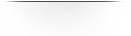Cost Risk Analysis
Cost is a driving consideration in decisions that determine how systems are developed, produced, and sustained.  Critical to these decisions is understanding how risk and uncertainty affects a system’s cost.  The process of identifying, measuring, and interpreting these effects is known as cost uncertainty analysis.  Used early, cost uncertainty analysis can expose potentially crippling areas of cost risk in systems.  This provides managers time to define and implement corrective strategies.  Moreover, the analysis brings realism to technical and managerial decisions that define a system’s overall engineering strategy.

Since the 1950s a substantial body of scholarship on cost risk analysis has evolved.  Published material appears in numerous industry and governmenttechnical reports, symposia proceedings, and professional journals.  Despite this, there is a need in the systems engineering community to synthesize prior scholarship and relate it to advances in technique and problem sophistication.  This book addresses that need.  It is a reference for systems engineers, cost engineers, management scientists, and operations research analysts.  It is also a text for students of these disciplines.

As a text, this book is appropriate for an upper-level undergraduate (or graduate-level) course on the application of probability methods to cost risk analysis problems.  It is assumed readers have a solid foundation in differential and integral calculus.  An introductory background in probability theory, as well as systems and cost engineering, is helpful; however, the important concepts are developed as needed. A rich set of theoretical and applied exercises accompanies each chapter.

Throughout the book, detailed discussions on issues associated with cost risk and cost uncertainty analysis are given.  This includes the treatment of correlation between the cost of various system elements, how to present the analysis to decision-makers, and the use of bivariate probability distributions to capture the joint interactions between cost and schedule.  Analytical techniques from probability theory are stressed, along with the Monte Carlo simulation method.  Numerous examples and case discussions are provided to illustrate the practical application of theoretical concepts.HomeProbability Methods...Analytical Methods...Advanced Risk Analysis